## 13.3.3 Motion of a Rigid Body

For a free-floating 3D rigid body, recall from Section 4.2.2 that its C-spacehas six dimensions. Suppose that actions are applied to the body as external forces. These directly cause accelerations that result in second-order differential equations. By defining a state to be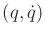, first-order differential equations can be obtained in a twelve-dimensional phase space.

Let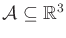denote a free-floating rigid body. Let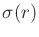denote the body density at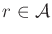. Let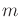denote the total mass of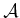, which is defined using the density as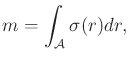(13.77)

in which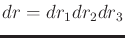represents a volume element in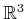. Let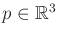denote the center of mass of, which is defined for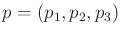as(13.78)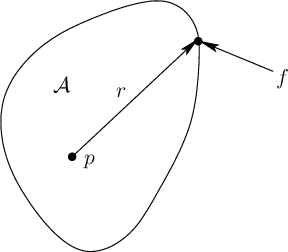Suppose that a collection of external forces acts on(it is assumed that all internal forces incancel each other out). Each force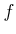acts at a point on the boundary, as shown in Figure 13.10 (note that any point along the line of force may alternatively be used). The set of forces can be combined into a single force and moment that both act about the center of mass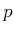. Let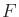denote the total external force acting on. Let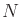denote the total external moment about the center of mass of. These are given by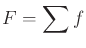(13.79)

and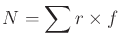(13.80)

for the collection of external forces. The termsandare often called the resultant force and resultant moment of a collection of forces. It was shown by Poinsot that every system of forces is equivalent to a single force and a moment parallel to the line of action of the force. The result is called a wrench, which is the force-based analog of a screw; see  for a nice discussion.

Actions of the form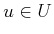can be expressed as external forces and/or moments that act on the rigid body. For example, a thruster may exert a force on the body when activated. For a given, the total force and moment can be resolved to obtain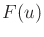and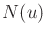.

Subsections
Steven M LaValle 2020-08-14